# 1 Introduction

DALEX explainers may be used to see what type of relation the model can learn / what the model has learned.

If we know the ground truth then we may verify model capability of learning particular types of relations.

# 2 Simulated data

Let’s simulate a model response as a function of four arguments

$(2x_1-1)^2 + sin(10 x_2) + x_3^{6} + (2 x_4 - 1) + |2x_5-1|$

set.seed(13)
N <- 250
X1 <- runif(N)
X2 <- runif(N)
X3 <- runif(N)
X4 <- runif(N)
X5 <- runif(N)

f <- function(x1, x2, x3, x4, x5) {
((x1-0.5)*2)^2-0.5 + sin(x2*10) + x3^6 + (x4-0.5)*2 + abs(2*x5-1)
}
y <- f(X1, X2, X3, X4, X5)

# 3 Model fits

Let’s compare four models: fandom forest, svm, lm and the ground truth.

library(randomForest)
library(DALEX)
library(e1071)
library(rms)

df <- data.frame(y, X1, X2, X3, X4, X5)

model_rf <- randomForest(y~., df)
model_svm <- svm(y ~ ., df)
model_lm <- lm(y ~ ., df)

# thanks to https://github.com/pbiecek/DALEX/issues/24
## important setup step required for use of rms functions
## add rcs terms to linear model
## this is a very convenient, objective way to account for non-linearity
## still a "linear" model because terms are linear combinations (additive)
model_rms <- ols(y ~ rcs(X1) + rcs(X2) + rcs(X3) + rcs(X4) + rcs(X5), df)

ex_rf <- explain(model_rf)
ex_svm <- explain(model_svm)
ex_lm <- explain(model_lm)
ex_rms <- explain(model_rms, label = "rms", data = df[, -1], y = df\$y)
ex_tr <- explain(model_lm, data = df[,-1],
predict_function = function(m, x) f(x[,1], x[,2], x[,3], x[,4], x[,5]),
label = "True Model")

# 4 Explainers

For X1 we want to see (2*x1 - 1)^2.

The linear model cannot guess the relation without prior preprocessing, the random forest is seeing something but the closest bet is from svm models.

library(ggplot2)
plot(single_variable(ex_rf, "X1"),
single_variable(ex_svm, "X1"),
single_variable(ex_lm, "X1"),
single_variable(ex_rms, "X1"),
single_variable(ex_tr, "X1")) +
ggtitle("Responses for X1. Truth: y ~ (2*x1 - 1)^2")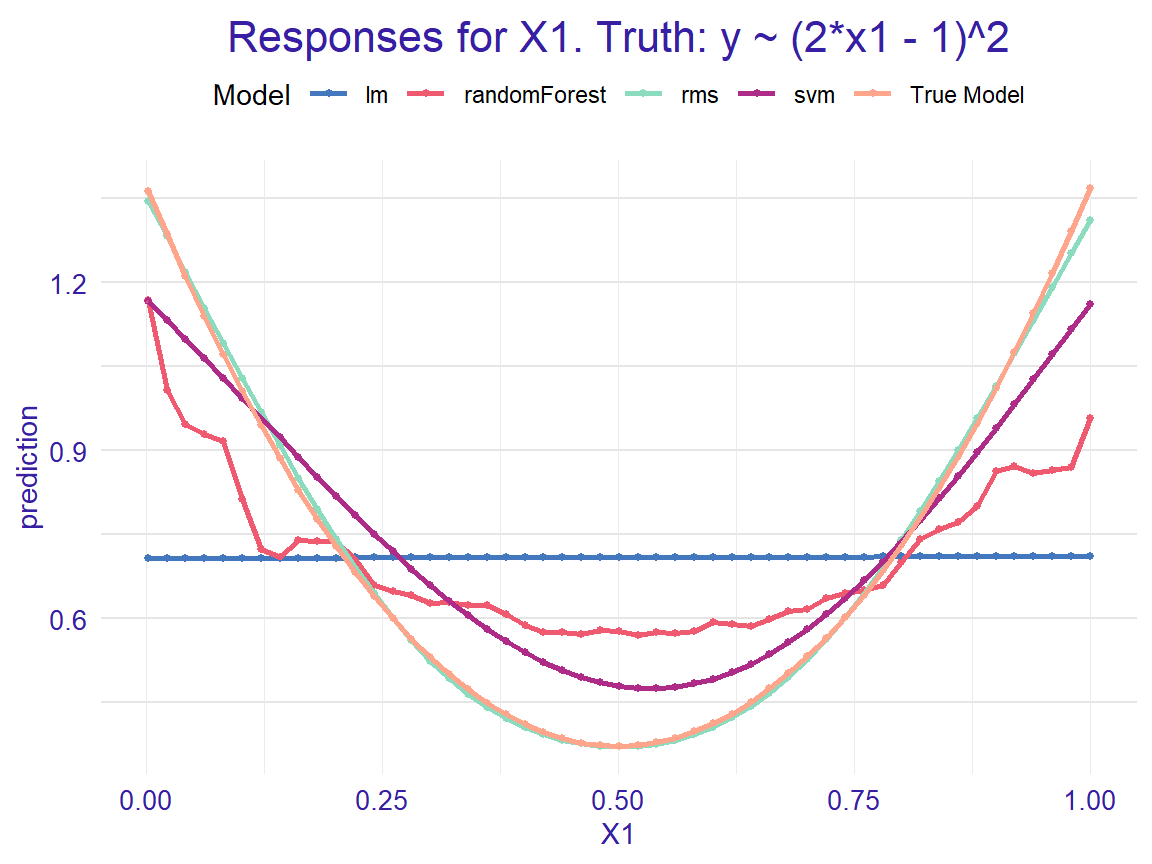For X2 we want to see sin(10 * x2).

The random forest guesses the shape, svm is not that elastic, the linear model does not see anything.

plot(single_variable(ex_rf, "X2"),
single_variable(ex_svm, "X2"),
single_variable(ex_lm, "X2"),
single_variable(ex_rms, "X2"),
single_variable(ex_tr, "X2")) +
ggtitle("Responses for X2. Truth: y ~ sin(10 * x2)")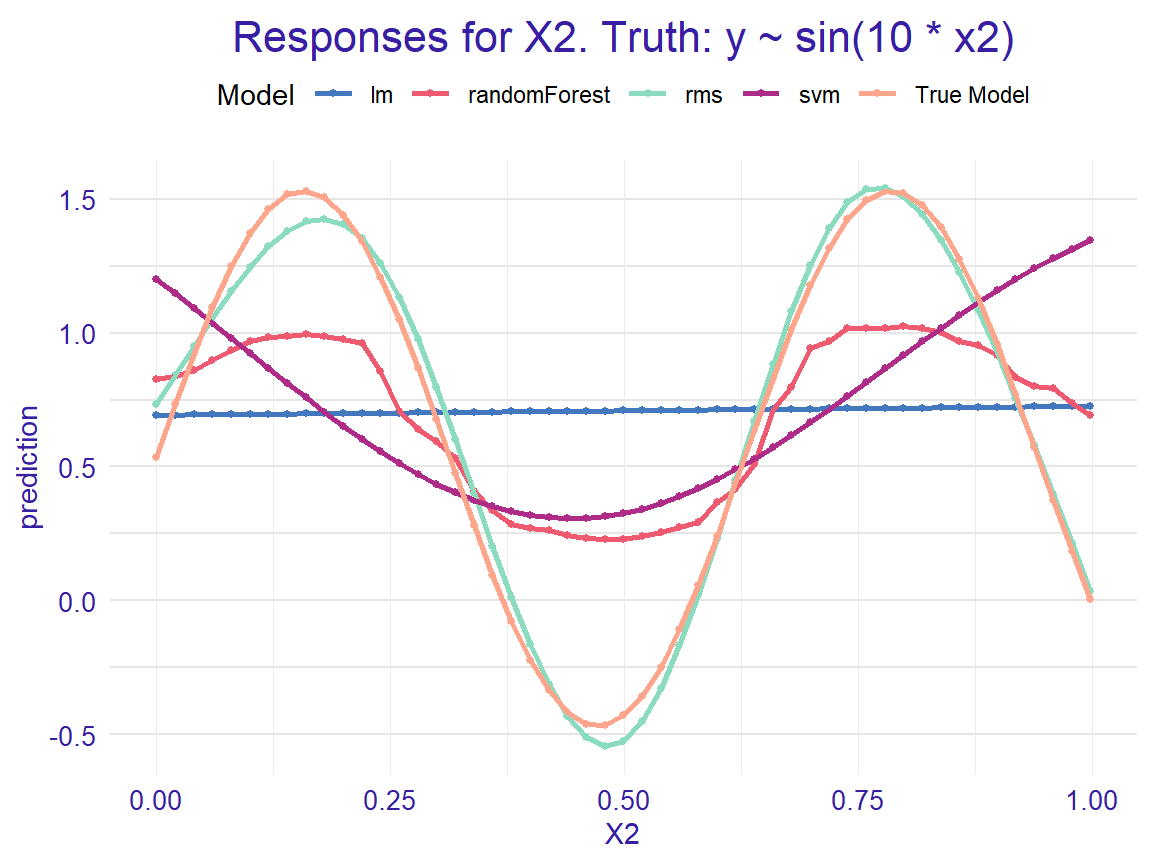For X3 we want to see x3^6.

The random forest is still able to guesses the shape, svm and linear are close.

plot(single_variable(ex_rf, "X3"),
single_variable(ex_svm, "X3"),
single_variable(ex_lm, "X3"),
single_variable(ex_rms, "X3"),
single_variable(ex_tr, "X3")) +
ggtitle("Responses for X3. Truth: y ~ x3^6")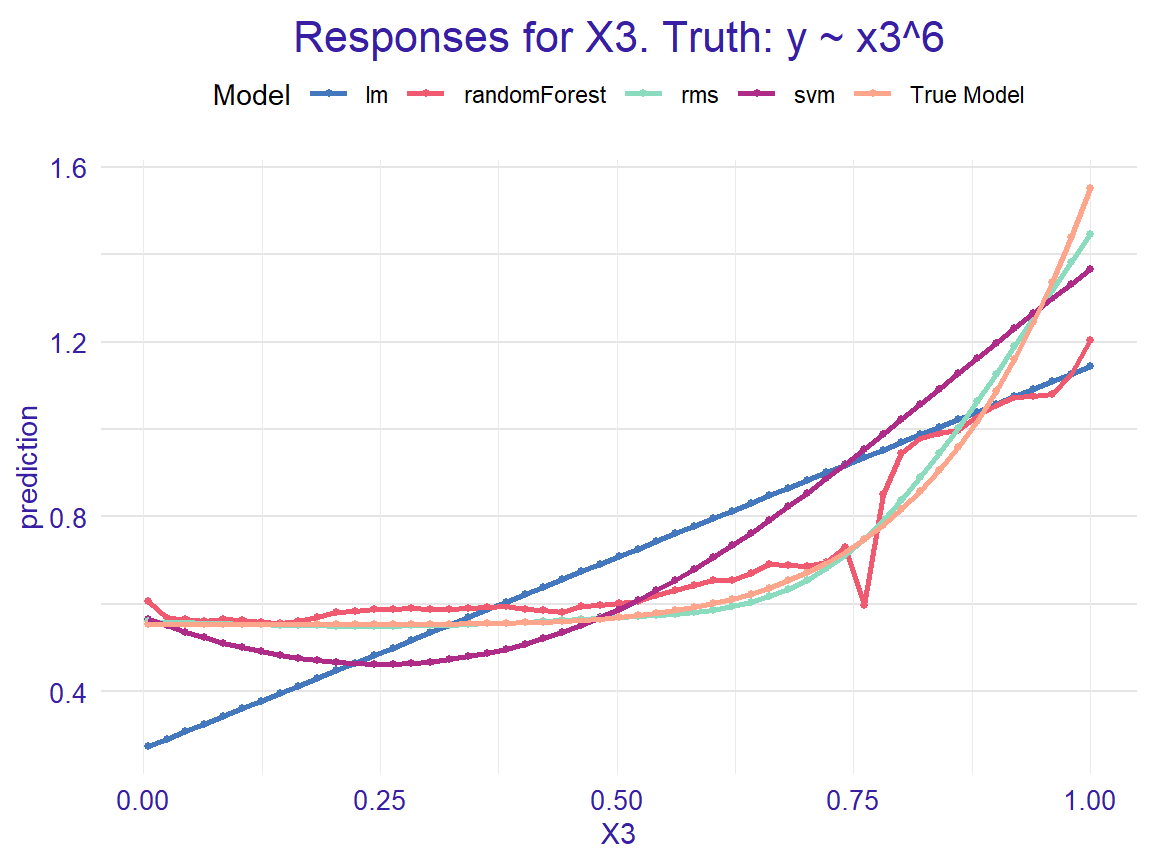For X4 we want to see 2 x4 - 1.

The linear model is doing the best job (as expected), svm are still pretty good, random forest model is more biased towards the mean.

plot(single_variable(ex_rf, "X4"),
single_variable(ex_svm, "X4"),
single_variable(ex_lm, "X4"),
single_variable(ex_rms, "X4"),
single_variable(ex_tr, "X4")) +
ggtitle("Responses for X4. Truth: y ~ (2 * x4 - 1)")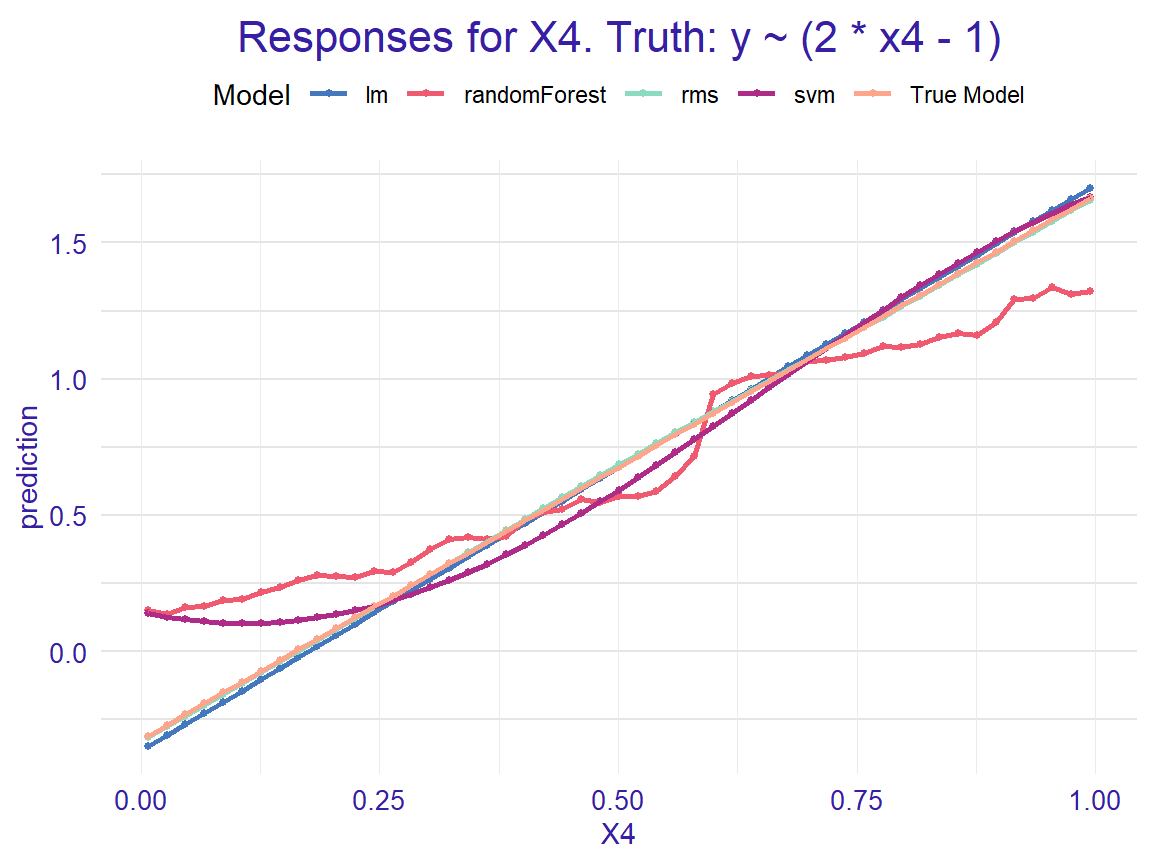For X5 we want to see |2 x5 - 1|.

All models except the lieanr one are guessing the shape.

plot(single_variable(ex_rf, "X5"),
single_variable(ex_svm, "X5"),
single_variable(ex_lm, "X5"),
single_variable(ex_rms, "X5"),
single_variable(ex_tr, "X5")) +
ggtitle("Responses for X5. Truth: y ~ |2 * x5 - 1|")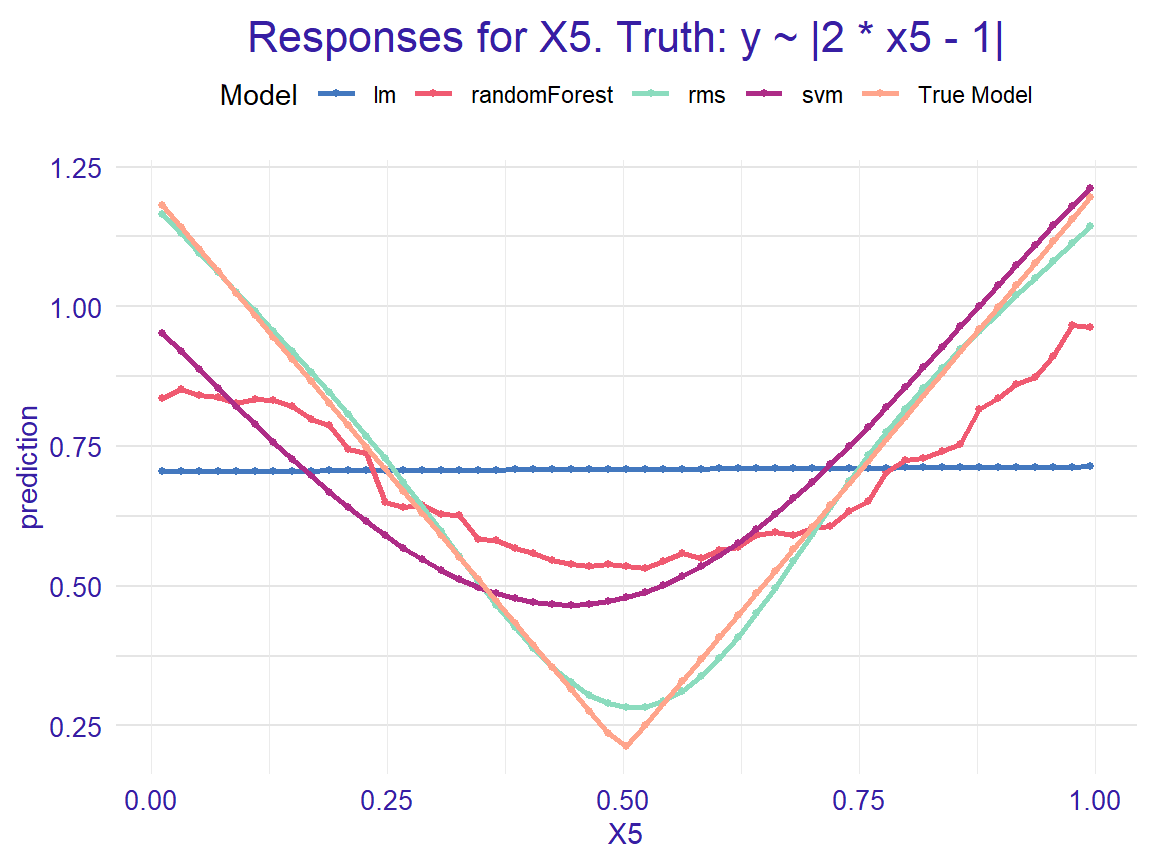sessionInfo()
## R version 3.6.1 (2019-07-05)
## Platform: x86_64-w64-mingw32/x64 (64-bit)
## Running under: Windows 10 x64 (build 17763)
##
## Matrix products: default
##
## locale:
##  LC_COLLATE=Polish_Poland.1250  LC_CTYPE=Polish_Poland.1250
##  LC_MONETARY=Polish_Poland.1250 LC_NUMERIC=C
##  LC_TIME=Polish_Poland.1250
##
## attached base packages:
##  stats     graphics  grDevices utils     datasets  methods   base
##
## other attached packages:
##   rms_5.1-3.1         SparseM_1.77        Hmisc_4.2-0
##   ggplot2_3.2.1       Formula_1.2-3       survival_2.44-1.1
##   lattice_0.20-38     e1071_1.7-2         DALEX_0.4.9
##  randomForest_4.6-14
##
## loaded via a namespace (and not attached):
##   Rcpp_1.0.3          mvtnorm_1.0-11      class_7.3-15
##   zoo_1.8-6           assertthat_0.2.1    digest_0.6.22
##   plyr_1.8.4          R6_2.4.0            backports_1.1.5
##  acepack_1.4.1       MatrixModels_0.4-1  evaluate_0.14
##  pillar_1.4.2        rlang_0.4.1         lazyeval_0.2.2
##  multcomp_1.4-10     rstudioapi_0.10     data.table_1.12.2
##  rpart_4.1-15        Matrix_1.2-17       checkmate_1.9.4
##  rmarkdown_1.15      labeling_0.3        splines_3.6.1
##  stringr_1.4.0       foreign_0.8-71      htmlwidgets_1.3
##  munsell_0.5.0       compiler_3.6.1      xfun_0.9
##  pkgconfig_2.0.3     base64enc_0.1-3     htmltools_0.3.6
##  nnet_7.3-12         tidyselect_0.2.5    tibble_2.1.3
##  gridExtra_2.3       htmlTable_1.13.1    codetools_0.2-16
##  crayon_1.3.4        dplyr_0.8.3         withr_2.1.2
##  MASS_7.3-51.4       grid_3.6.1          nlme_3.1-140
##  polspline_1.1.15    gtable_0.3.0        magrittr_1.5
##  scales_1.0.0        stringi_1.4.3       latticeExtra_0.6-28
##  sandwich_2.5-1      TH.data_1.0-10      RColorBrewer_1.1-2
##  tools_3.6.1         pdp_0.7.0           glue_1.3.1
##  purrr_0.3.3         yaml_2.2.0          colorspace_1.4-1
##  cluster_2.1.0       knitr_1.24          quantreg_5.51